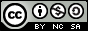- 目录 -
LeetCode 面试题51 数组中的逆序对(线段树+树状数组+离散化)

输入: [7,5,6,4]



0 <= 数组长度 <= 50000


## 代码

  1 2 3 4 5 6 7 8 9 10 11 12 13 14 15 16 17 18 19 20 21 22 23 24 25 26 27 28 29 30 31 32 33 34 35 36 37 38 39 40 41 42 43 44 45 46 47 48 49 50 51 52 53 54 55 56 57 58 59 60 61 62 63 64  class Solution { public: int sum[4 * 50001]; void pushup(int rt) { sum[rt] = sum[rt << 1] + sum[rt << 1 | 1]; } void build(int l, int r, int rt) { sum[rt] = 0; if (l == r) return; int m = (l + r) >> 1; build(l, m, rt << 1); build(m + 1, r, rt << 1 | 1); } void update(int p, int l, int r, int rt) { if (l == r) { sum[rt]++; return; } int m = (l + r) >> 1; if (p <= m) update(p, l, m, rt << 1); else update(p, m + 1, r, rt << 1 | 1); pushup(rt); } int query(int L, int R, int l, int r, int rt) { if (L <= l && r <= R) { return sum[rt]; } int m = (l + r) >> 1; int ret = 0; if (L <= m) ret += query(L, R, l, m, rt << 1); if (R > m) ret += query(L, R, m + 1, r, rt << 1 | 1); return ret; } int reversePairs(vector &nums) { if (nums.size() == 0) return 0; vector temp(nums); sort(temp.begin(), temp.end()); temp.erase(unique(temp.begin(), temp.end()), temp.end()); build(1, temp.size(), 1); int cnt = 0; for (int i = 0; i < nums.size(); i++) { int l = lower_bound(temp.begin(), temp.end(), nums[i]) - temp.begin() + 1; int r = temp.size(); cnt += query(l + 1, r, 1, r, 1); update(l, 1, r, 1); } return cnt; } }; 

  1 2 3 4 5 6 7 8 9 10 11 12 13 14 15 16 17 18 19 20 21 22 23 24 25 26 27 28 29 30 31 32 33 34 35 36 37 38 39 40 41 42 43 44 45 46 47 48 49  class Solution { public: int c[50000 + 1], N; void init(int n) { memset(c, 0, sizeof(0)); N = n; } int lowbit(int x) { return x & -x; } void add(int i, int k) { while (i <= N) { c[i] += k; i += lowbit(i); } } int sum(int n) { int sum = 0; while (n > 0) { sum += c[n]; n -= lowbit(n); } return sum; } int reversePairs(vector &nums) { if (nums.size() == 0) return 0; vector temp(nums); sort(temp.begin(), temp.end()); temp.erase(unique(temp.begin(), temp.end()), temp.end()); init(temp.size()); int cnt = 0; for (int i = 0; i < nums.size(); i++) { int x = lower_bound(temp.begin(), temp.end(), nums[i]) - temp.begin() + 1; add(x, 1); cnt += i + 1 - sum(x); } return cnt; } };